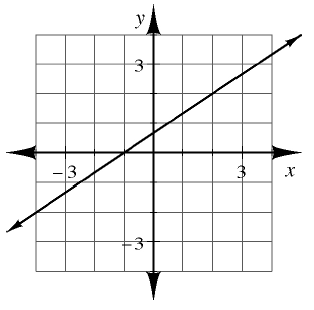### Home > GB8I > Chapter 4 Unit 5 > Lesson INT1: 4.1.4 > Problem4-39

4-39.

Multiple Choice: Martha’s equation has the graph shown at right. Which of these are solutions to Martha’s equation? (Remember that more than one answer may be correct.)Which of the given ordered pairs is on the line?

1.  $\quad ( - 4 , - 2 )$

1.  $( - 1,0 )$

1.  $x = 0 \text { and } y = 1$

1.  $x = 2 \text { and } y = 2$